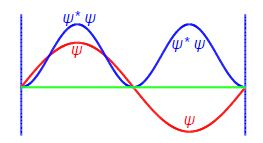# Quantum Chemistry

Question: In the quantum mechanics of electrons, atoms, molecules, or ions; chemists make use of wavefunctions ψ. The reason is that the value of the wavefunction often varies in a cyclic fashion and so can very naturally be expressed as a complex number. If the wavefunction ψ has the value

Ψ = A + i B

the probability of the system having that value of ψ is related to the product of the complex conjugate ψ* and the wavefunction itself ψ* ψ. Find whether the probability of the system is a real number?

Solution:

Multiplying the wavefunction by its complex conjugate is easily done with basic complex number maths.The product of  ψ* ψ is always real and always positive (≥ 0), i.e. a wavefunction probability at a given point in space will be a real positive number, as it should be if the concept of probability is to make sense. Below Figure shows the wavefunction (red) for the n = 2 quantum level of the particle in a 1-dimensional box is both a negative and positive complex number where the green line is the zero level for both ψ2 and ψ*2 ψ2. The probability of the particle being at a certain position (blue) in the box is a positive real number.